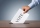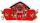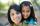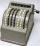Drive

At the bus stop 8 people take out and 10 people take into bus. Next stop 6 take out and 5 take in. On the third stop 6 take out and 3 take in. The bus traveled further with 39 people.

How many passengers were originally at the bus?

Result

n =  41

Solution:Leave us a comment of this math problem and its solution (i.e. if it is still somewhat unclear...):Be the first to comment!To solve this verbal math problem are needed these knowledge from mathematics:

Do you have a linear equation or system of equations and looking for its solution? Or do you have quadratic equation?

Next similar math problems:

1. Flood waterFlood waters in some US village meant that the homes had to evacuate 364 people. 50 of them stayed at elementary schools, 59 them slept with their friends and others went to relatives. How many people have gone to relatives?
2. Voting400 employees cast their votes in a board member election that has only 2 candidates. 120 people vote for candidate A, while half of the remaining voters abstain. How many votes does candidate B receive?
3. The rodThe rod has a length of 90cm. Half is painted blue, the third is red and the rest is yellow. How long is the yellow part of the rod?
4. Forest nurseryIn the forest nursery after winter, they found that 1/10 stems died out of them. For them, they land 193 new spruces. How many spruces are in the forest nursery?
5. Addition of Roman numbersAdded together and write as decimal number: LXVII + MLXIV
6. Roman numerals 2+Add up the number writtens in Roman numerals. Write the results as a roman numbers.
7. If-then equationIf 5x - 17 = -x + 7, then x =
8. Six te 2If 3t-7=5t, then 6t=
9. Eq1Solve equation: 4(a-3)=3(2a-5)
10. Simple equation 8Solve the following equation: 36=-(1+7x)-6(-7-x)
11. Roman numerals +Add up the number writtens in Roman numerals. Write the results as a decimal number.
12. I think numberI think number.When I add 841 to it and subtract 157, I get a number that is 22 greater than 996. What number I thinking?
13. Brother and sisterWhen I was 8, my sister was 4, now I am 18, how old is my sister?
14. PupilsTwo classes to collect money. Boys are four sevenths pupils. In time did not pay a quarter of the boys and a sixth of girls, which together mean 12 sinners. How many pupils attend this two classes ?
15. Expression 1What is 7+8-(5×2)+5-4+(6×(5-3)+6)-(8+10)-7+6?
16. Mom and daughterMom is 30 years older than the daughter. What is the age difference between them in 35 years?
17. OperationsSum of the numbers 1.01 and 3.35 multiply by the difference of numbers 6.69 and 1.39.